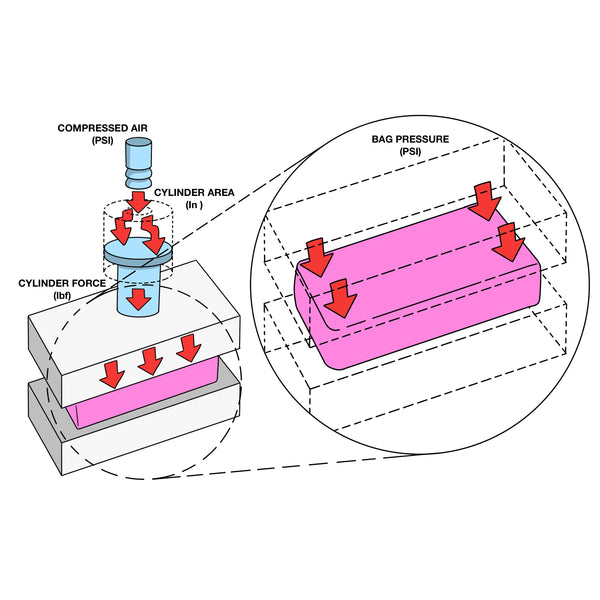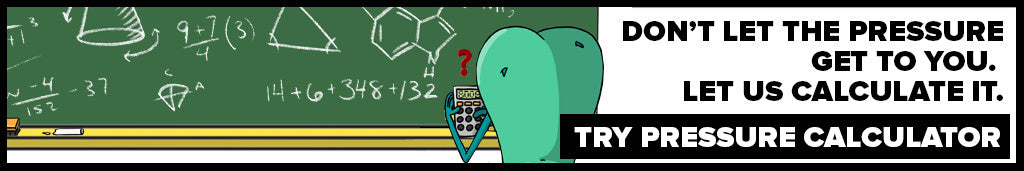# How to Calculate Platen PSI### Viviane Schute

Cannabis enthusiast and student of the art of solventless extraction

✅ Updated 3/4/21

Achieving the correct level of pressure during solventless extraction frees cannabis to unlock that unique expression of cannabinoid and terpene-rich oil we know and love as rosin. So how is pressure measured, and how can I know the actual amount of pressure inside my rosin bag?

Pressing high grade rosin requires exerting just the right amount of pressure onto cannabis source material. Too much pressure and you risk equipment failure and reduced oil flow efficiency. Too little pressure and you end up overcompensating with excessive temperatures and evaporating away terpenes.

How Is Pressure Measured?

The level of pressure we need to control during extraction is NOT the same thing as how many tons of force we apply from the press itself onto the rosin plates. The pressure we want to measure isn’t given by internal pressure gauges on DIY rosin presses.

Measuring the pressure exerted on your cannabis starting material as pounds per square inch (PSI) is the most effective way to convey actual pressure generated during rosin extraction. Measuring PSI at the rosin bag itself, often referred to as platen PSI, gives us the clearest reading of actual pressure on your source material inside the rosin bag.

Calculating platen PSI requires a measurement of the dimensions of your rosin bag (area in square inches) and the tonnes of force being applied (in pounds).

Platen pressure tells us how much pressure is being exerted onto the source material. This pressure onto the source material is the only pressure that matters during extraction.

Platen pressure equals the pounds of force divided by the surface area of your source material, which closely matches the rosin bag. Stated another way, Platen PSI is the relationship between the dimensions of your source material and the tones of force being applied.

The area of your rosin bag is a simple length x width calculation. The pounds of force exerted may require a couple additional steps, depending on the type of press you're using and its internal gauge.Here is the Platen PSI calculator:

Platen PSI (Pressure on Source Material) = Force Exerted by Press / Source Material Surface Area

Calculating Force

There are different calculations of force generated by your press depending on whether the gauge on your press reads in tons (hydraulic press) or PSI (pneumatic press).

Hydraulic Presses (Output in Tons)
In most hydraulic shop presses, the internal pressure gauge is already matched to the diameter of the driving ram, so no additional calculations of force are needed. Check to make sure the ram diameter matches that of the gauge, and in this case no additional calculations of force are needed. We can use the tons of force on the gauge for our Platen PSI calculator.

Then we convert the tons into pounds. 1 ton = 2000 pounds. We’ll go through an example below.Pneumatic Presses (Output in PSI)
If you’re using a pneumatic press that displays internal pressure (PSI) on the gauge instead of  tones (force), then a conversion from pressure to force is required. If your press displays PSI on the pressure gauge, then do the following calculation to find the force being generated:

Force = PSI Reading on the Gauge x Cylinder's Effective Surface Area (The Rosin Press Ram)

The Cylinder's Effective Surface Area = 3.14 x Radius of Cylinder^2

Calculating Rosin Bag Surface Area

Now with the hard part over with, we move on to a more simple calculation, which is the surface area of the rosin bag, measured in inches.

Source Material Surface Area = Approximate length of the Bag x Approximate Width of the Bag

The dimensions we need are of the surface area of the source material, not necessarily the surface area of the rosin bag itself. So for example, if you’re using 4-inch bags but only filling the first 3 inches with source material, then the length you want to use for the Platen PSI calculation is 3 inches.

Similarly, there may be some adjustment due to the seams of the bag. Measure the surface area occupied by the cannabis, not the material of the filter bag itself.

Now we have everything that we need for the equation:Platen PSI (Pressure on Source Material) = Force Exerted by Press / Source Material Surface Area

Remember, the method of calculating force will vary depending on the press you’re using, either hydraulic (gauge reading in tons and matched to the cylinder) or pneumatic (gauge reading in psi). Let's go through a couple examples.

Simple Example - The Hydraulic Press

Press: H-Frame hydraulic press with gauge matched to cylinder  Force: 2 tons on gauge during extraction
Rosin Bags: The Press Club’s 2x4in in rosin bags

First convert tons to pounds. 2 tons = 4000 lbs

Platen PSI = Force (4000 lbs)  / Rosin Bag Surface Area (1.95 x 3.2in)

Note the rosin bag surface area is not 2x4 inches because we adjusted for width of seams and length of the bag actually filled with cannabis. These adjustments brought the dimensions to 1.95 x 3.2 inches, which is the surface area of the rosin bag occupied by the cannabis.

Platen PSI = 4000 lbs / 6.24 Inches Squared = 641 PSI

More Complex Example - The Pneumatic Press

Press: Pneumatic press with gauge measuring PSI, cylinder with radius 2 inches
Force: 250 PSI being displayed on pressure gauge during extraction
Rosin Bags: The Press Club’s 2x4in in rosin bags

Platen PSI = Force / Source Material Surface Area

Force = 250 PSI x (3.14 x 2^2) = 3140 lbs

Source Material (Rosin Bag) Surface Area = 1.95 x 3.2 = 6.24 Inches Squared

Our pressure at the rosin bag in this example = 3140 / 6.24 = 503 PSIHow Much Pressure Do I Need?

How much pressure to apply depends in large part on the source material you’re using. It’s generally recommended that bubble hash is pressed at a lower PSI than flower. The ranges we recommend are:

Flower: 1000-2000 PSI
Bubble Hash: 500 - 1500 PSI
Dry Sift: 500 - 1500 PSI

There are other factors to weigh in your decision on pressure applied during extraction. Go more in depth into the topic with our Rosin Press Pressure Guide

Conclusion

Knowing the amount of pressure applied to source material during extraction is much more powerful than simply knowing the tons of force being exerted, or the PSI of the press itself. This is because it allows us to measure changes in the pressure being applied to source material as a correlation with adjustments in the size of our rosin bags, or even how much we’re filling them. These variables should not be overlooked, otherwise we’re guessing on the total pressure we’re actually harnessing during extraction.

Now that you know how to calculate your own platen PSI, you can better measure and control this critically important variable in the extraction process.

Press on!

Thoughts? Let us know by joining our secret Facebook group. Hang out with a community of like-minded solventless heads like yourself. Ask our head extractor questions, share your latest press and learn from hobbyists and experts in the industry.

What is Platen psi?
Platen PSI is the amount of pressure that's being applied to cannabis material inside the rosin filter bag. This is not the same thing as the amount of pressure being generated internally by the press itself.

What does PSI stand for?
PSI stands for pounds per square inch, which is a measurement of pressure.

How do you calculate Platen PSI?
The formula for calculating platen PSI is Force Exerted by Rosin Press divided by Source Material Surface Area. The calculation of force exerted by your rosin press varies depending on what type of rosin press you're using.

Is force different than pressure?
Yes, force is different than pressure. Force is the amount of weight exerted by the rosin press, while pressure is a function of that weight applied over a specific surface area. Force is measured in tons, while pressure is measured in PSI (pounds per square inch).

Is the reading on my rosin press the same thing as Platen PSI?
No, the reading on your rosin press is not the same thing as the Platen PSI. We are concerned about the Platen PSI, not the reading on your rosin press. We need to divide the force generated by your rosin press by the surface area of the rosin filter bag you're using.G Lawrence

Everything on pressure makes sense and I understand the calculations. The problem is the bottle presses don’t have a PSI gauge? Is there a way to calculate by cranks of the handle?

ColpittsCraftCannaProducts

Great information. This information needs to be more readily available. I see too many mistakes being made just from not understanding platen psi. I see a lot of people trying to press quality products without any understanding of what they are doing. Like trying to press hash at 12 to 15 tons of pressure. Anyways… Thank you.